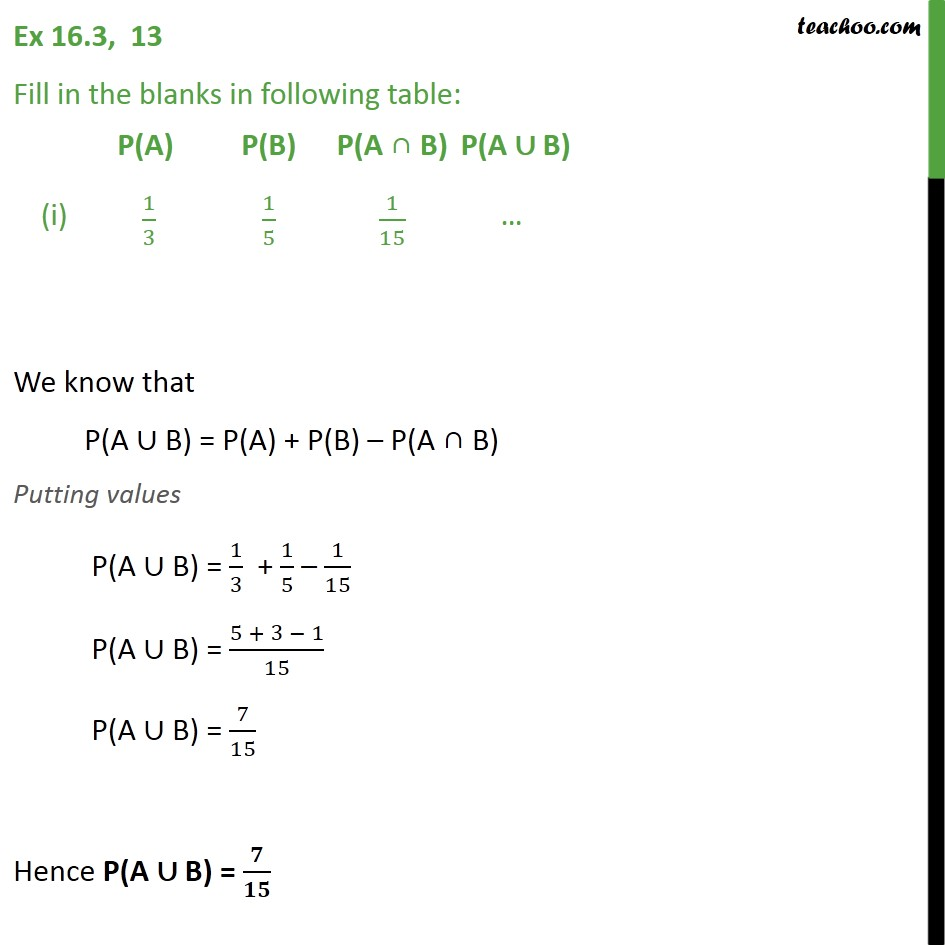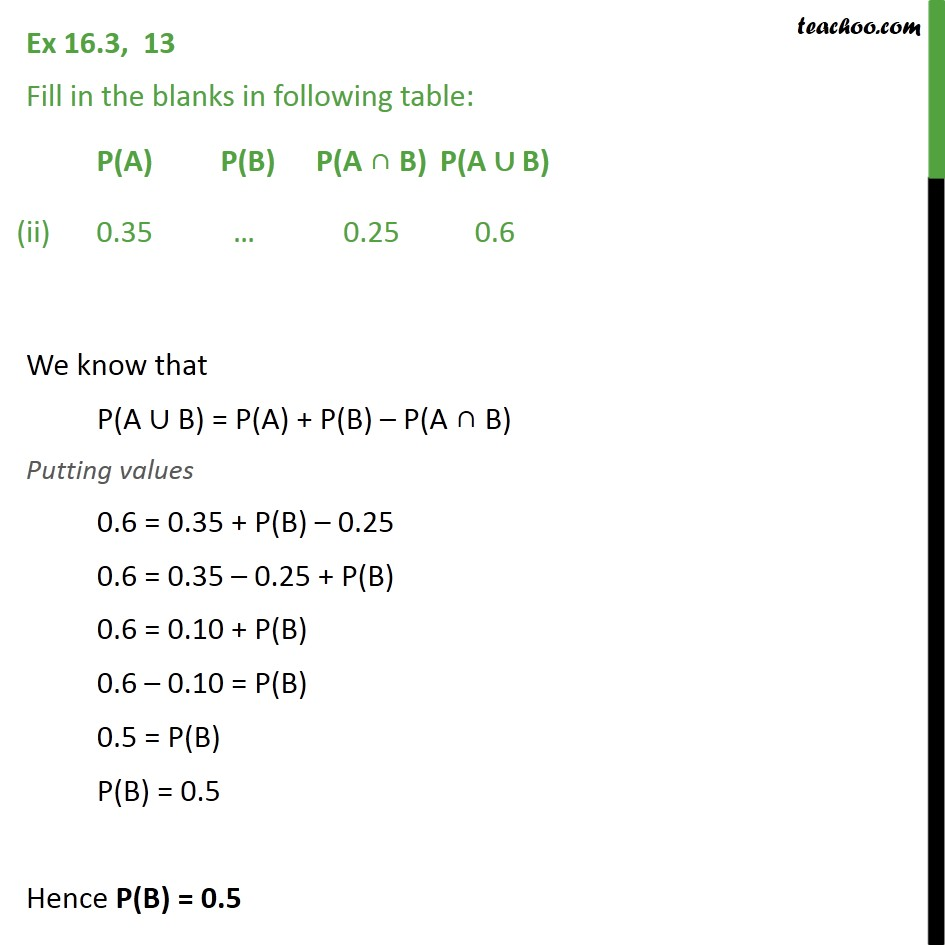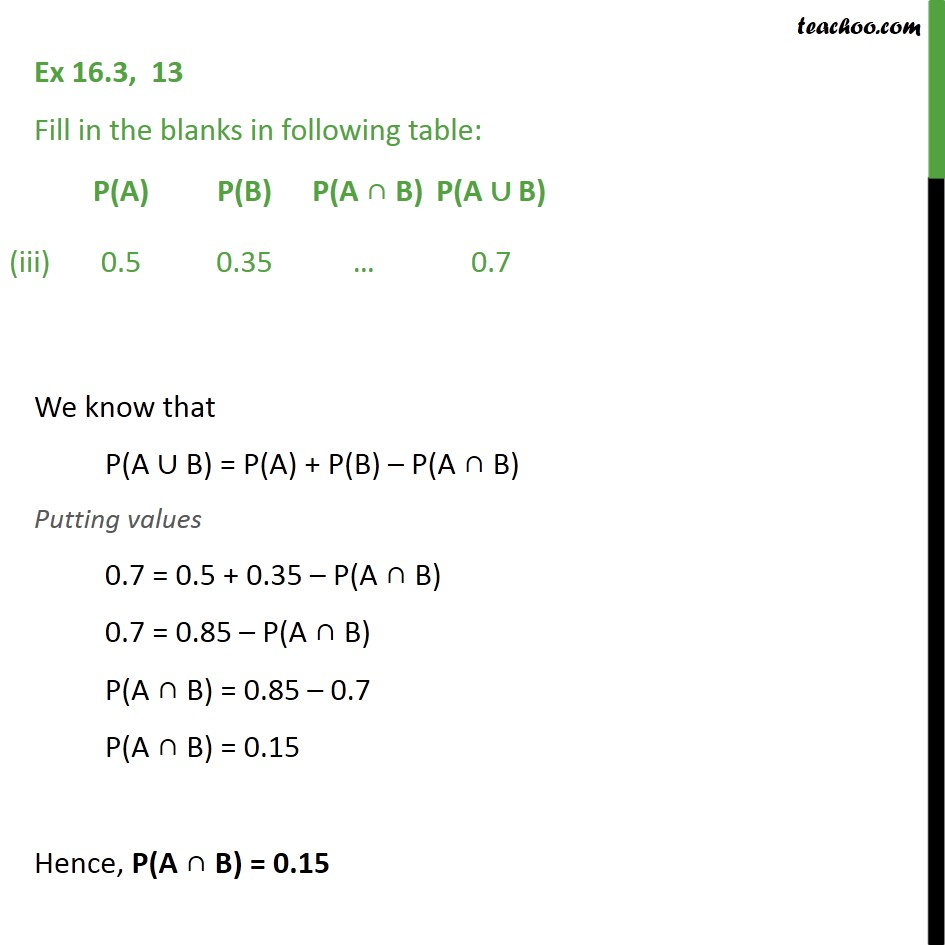Using formulae of sets

Chapter 16 Class 11 Probability
Concept wiseIntroducing your new favourite teacher - Teachoo Black, at only ₹83 per month

### Transcript

Ex 16.3, 13 Fill in the blanks in following table: We know that P(A ∪ B) = P(A) + P(B) – P(A ∩ B) Putting values P(A ∪ B) = 1﷮3﷯ + 1﷮5﷯ – 1﷮15﷯ P(A ∪ B) = 5 + 3 − 1﷮15﷯ P(A ∪ B) = 7﷮15﷯ Hence P(A ∪ B) = 𝟕﷮𝟏𝟓﷯ Ex 16.3, 13 Fill in the blanks in following table: We know that P(A ∪ B) = P(A) + P(B) – P(A ∩ B) Putting values 0.6 = 0.35 + P(B) – 0.25 0.6 = 0.35 – 0.25 + P(B) 0.6 = 0.10 + P(B) 0.6 – 0.10 = P(B) 0.5 = P(B) P(B) = 0.5 Hence P(B) = 0.5 Ex 16.3, 13 Fill in the blanks in following table: We know that P(A ∪ B) = P(A) + P(B) – P(A ∩ B) Putting values 0.7 = 0.5 + 0.35 – P(A ∩ B) 0.7 = 0.85 – P(A ∩ B) P(A ∩ B) = 0.85 – 0.7 P(A ∩ B) = 0.15 Hence, P(A ∩ B) = 0.15